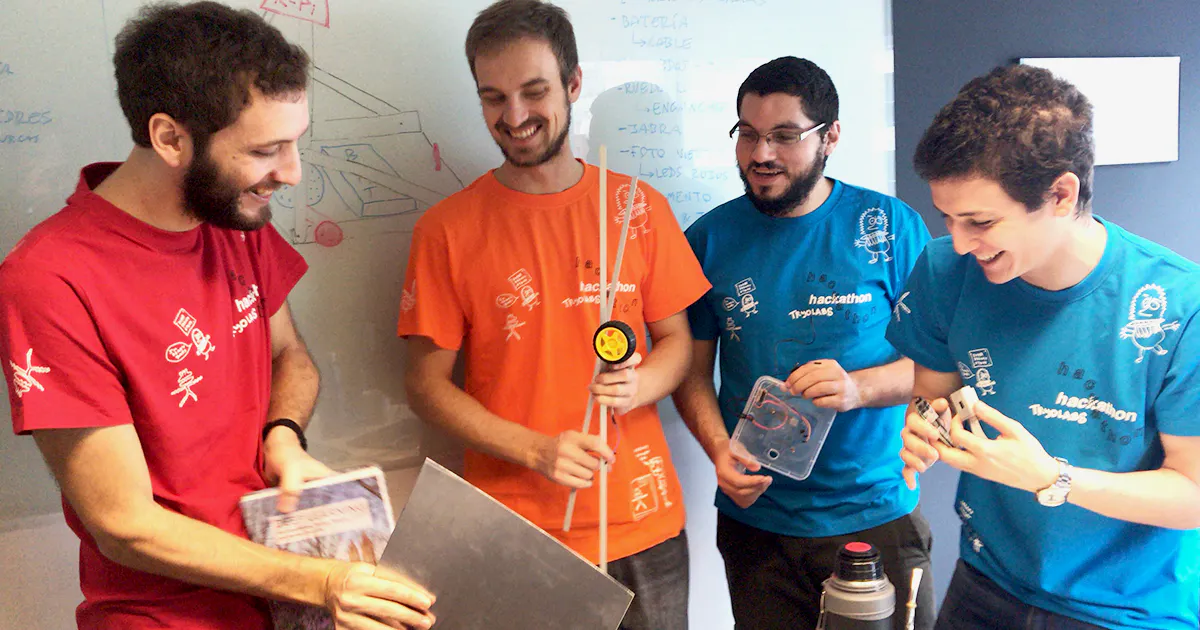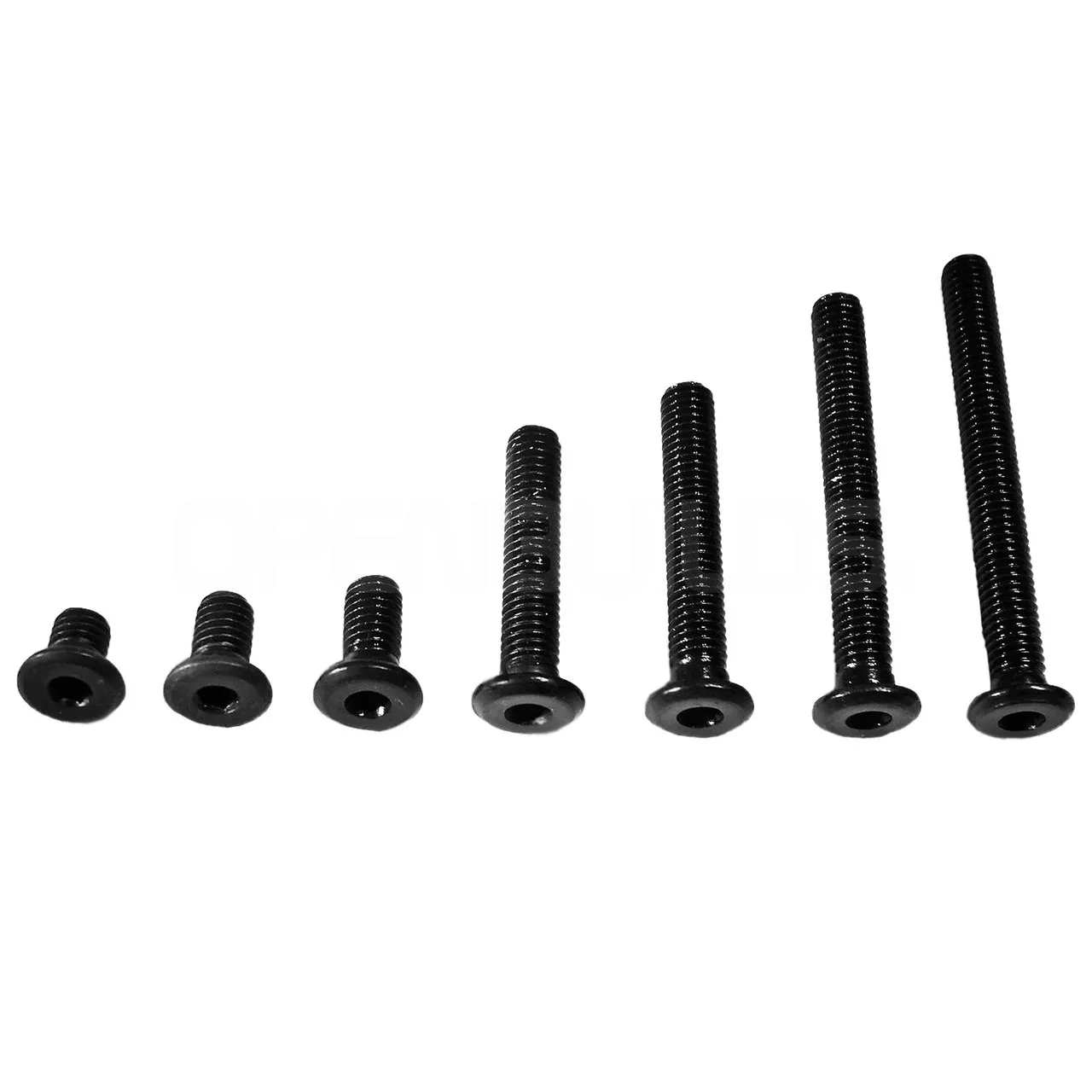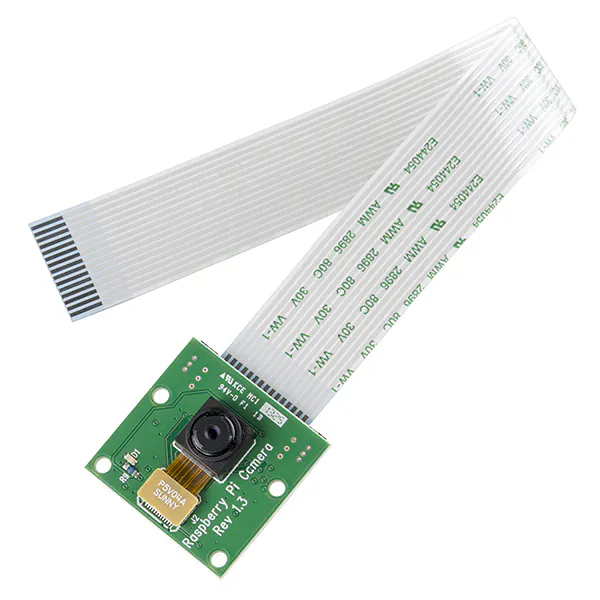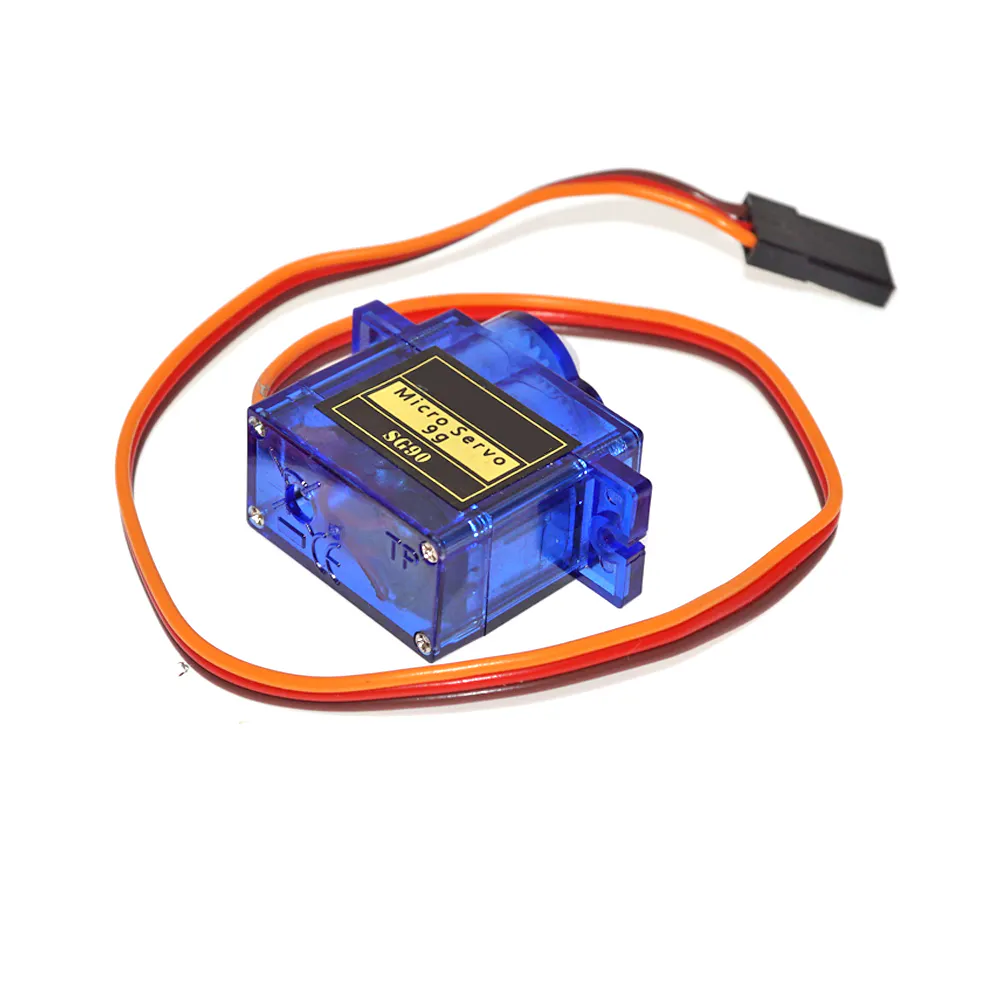Published

# Using IoT to Build a Stand-in Robot for Remote Collaboration

This is how we went on a mission to solve one of the biggest challenges of remote collaboration using IoT and computer vision.

IntermediateFull instructions provided8 hours685## Things used in this project

### Hardware components

 Layers of aluminium sheets
×1OpenBuilds Low Profile Screws M5 (10 Pack)
×1OpenBuilds Nylon Insert Hex Locknut - M5
×1Raspberry Pi 3 Model B
×1Texas Instruments Dual H-Bridge motor drivers L293D
×1Raspberry Pi Camera Module
×1SG90 Micro-servo motor
×1
 Tire wheel with DC motor and reducer
×2
 Swivel Caster
×1Jumper wires (generic)
×1
 Jabra Speaker
×1

### Software apps and online services

 TensorFlow PoseNet
 UV4L
 WebRTC extension for UV4L

## Code

### Code snippet #1

Plain text
```class MotorsWheels:

def __init__(
self, r_wheel_forward=6, r_wheel_backward=13, l_wheel_forward=19, l_wheel_backward=26):
self.r_wheel_forward = r_wheel_forward
...
GPIO.setmode(GPIO.BCM)
GPIO.setup(r_wheel_forward, GPIO.OUT)
GPIO.setup(r_wheel_backward, GPIO.OUT)
...
# Turn all motors off
GPIO.output(r_wheel_forward, GPIO.LOW)
GPIO.output(r_wheel_backward, GPIO.LOW)

def _spin_right_wheel_forward(self):
GPIO.output(self.r_wheel_forward, GPIO.HIGH)
GPIO.output(self.r_wheel_backward, GPIO.LOW)

def _stop_right_wheel(self):
GPIO.output(self.r_wheel_backward, GPIO.LOW)
GPIO.output(self.r_wheel_forward, GPIO.LOW)

def go_fw(self):
self._spin_left_wheel_forward()
self._spin_right_wheel_forward()

class ServoCamera:
CENTER = 40000
UP_LIMIT = 80000
DOWN_LIMIT = 30000
STEP = 5000

def __init__(self, servo=18, freq=50):
self.servo = servo
self.freq = freq
self.pi = pigpio.pi()

self.angle = self.CENTER
self._set_angle()

def _set_angle(self):
self.pi.hardware_PWM(self.servo, self.freq, self.angle)

def up(self):
if self.angle + self.STEP < self.UP_LIMIT:
self.angle += self.STEP
self._set_angle()

def down(self):
if self.angle - self.STEP > self.DOWN_LIMIT:
self.angle -= self.STEP
self._set_angle()
```

### Code snippet #2

Plain text
```class MotorsWheels:

def __init__(
self, r_wheel_forward=6, r_wheel_backward=13, l_wheel_forward=19, l_wheel_backward=26):
self.r_wheel_forward = r_wheel_forward
...
GPIO.setmode(GPIO.BCM)
GPIO.setup(r_wheel_forward, GPIO.OUT)
GPIO.setup(r_wheel_backward, GPIO.OUT)
...
# Turn all motors off
GPIO.output(r_wheel_forward, GPIO.LOW)
GPIO.output(r_wheel_backward, GPIO.LOW)

def _spin_right_wheel_forward(self):
GPIO.output(self.r_wheel_forward, GPIO.HIGH)
GPIO.output(self.r_wheel_backward, GPIO.LOW)

def _stop_right_wheel(self):
GPIO.output(self.r_wheel_backward, GPIO.LOW)
GPIO.output(self.r_wheel_forward, GPIO.LOW)

def go_fw(self):
self._spin_left_wheel_forward()
self._spin_right_wheel_forward()

class ServoCamera:
CENTER = 40000
UP_LIMIT = 80000
DOWN_LIMIT = 30000
STEP = 5000

def __init__(self, servo=18, freq=50):
self.servo = servo
self.freq = freq
self.pi = pigpio.pi()

self.angle = self.CENTER
self._set_angle()

def _set_angle(self):
self.pi.hardware_PWM(self.servo, self.freq, self.angle)

def up(self):
if self.angle + self.STEP < self.UP_LIMIT:
self.angle += self.STEP
self._set_angle()

def down(self):
if self.angle - self.STEP > self.DOWN_LIMIT:
self.angle -= self.STEP
self._set_angle()
```

### Code snippet #3

Plain text
```async function detectBody(canvas, net) {
if (net){
var ctx = canvas.getContext('2d');
var imageElement = ctx.getImageData(0, 0, canvas.width, canvas.height);

var imageScaleFactor = 0.3;
var flipHorizontal = false;
var outputStride = 16;
var maxPoseDetections = 2;
var poses = await net.estimateMultiplePoses(
imageElement,
imageScaleFactor,
flipHorizontal,
outputStride,
maxPoseDetections
)
return poses;
}
```

### Code snippet #4

Plain text
```async function detectBody(canvas, net) {
if (net){
var ctx = canvas.getContext('2d');
var imageElement = ctx.getImageData(0, 0, canvas.width, canvas.height);

var imageScaleFactor = 0.3;
var flipHorizontal = false;
var outputStride = 16;
var maxPoseDetections = 2;
var poses = await net.estimateMultiplePoses(
imageElement,
imageScaleFactor,
flipHorizontal,
outputStride,
maxPoseDetections
)
return poses;
}
```

### Github

https://github.com/tryolabs/vierjavibot

## Credits

### Tryolabs Team

1 project • 6 followers
We're a team of Machine Learning, Computer Vision & IoT specialists that likes sharing cool projects with the community.